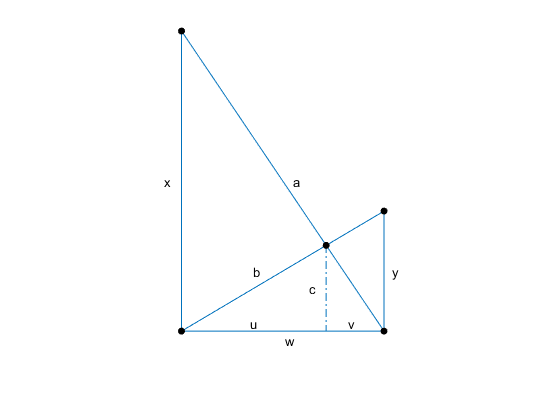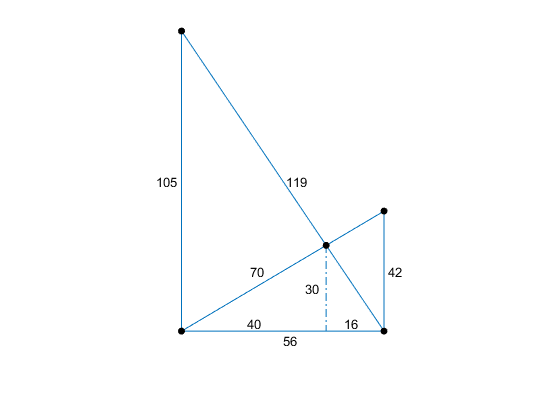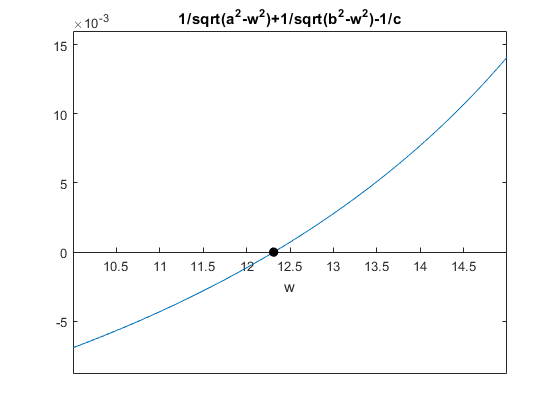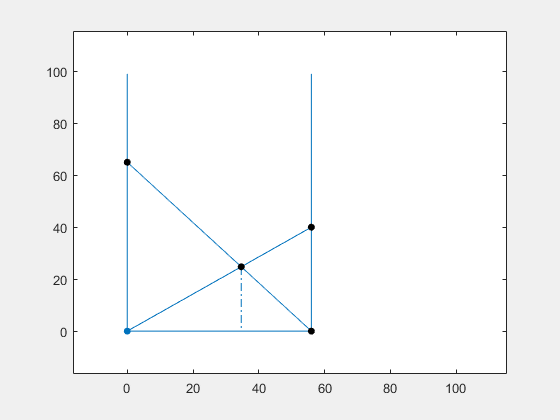# The Classic Crossed Ladders Puzzle

Here is a classic puzzle. A pair of ladders leaning against the sides of an alley form a lopsided cross. Each ladder is propped against the base of one wall and leans against the opposite wall. If one ladder is 30 feet long, the other 20 feet long, and the point where they cross 10 feet above the ground, how wide is the alley?

### Contents

#### Eight variables

The lengths of the ladders are denoted by $a$ and $b$. The height of the crossing is $c$. The heights of the points where the ladders reach the walls are $x$ and $y$. The bases of the right triangles whose common height is the crossing are $u$ and $v$. Finally, the width of the alley is $w$. Here is the picture.

   ladders_diagram('vars')#### The equations

These eight variables are connected by five equations. The Pythagorean Theorem provides two equations.

$$a^2 = x^2 + w^2$$

$$b^2 = y^2 + w^2$$

The ratios between sides of similar triangles provide two more.

$$\frac{x}{w} = \frac{c}{v}$$

$$\frac{y}{w} = \frac{c}{u}$$

The fifth equation is a simple linear relation that ties the two triangles together.

$$w = u + v$$

Five equations involving eight variables leaves three degrees of freedom. In principle we could specify any three of the variables and solve for the other five. But that may or may not be easy. We will have two different situations in what follows. The puzzle posed in the opening paragraph specifies $a$, $b$ and $c$, and asks to find $w$. This is nontrivial. On the other hand, our graphic app is driven by $x$, $y$ and $w$. When these three are pinned down by mouse clicks, the other five follow immediately.

#### Harmonic mean

Combining the last three equations generates an elegant relation. This isn't a new equation; it's a consequence of the ones we already have.

$$\frac{1}{c} = \frac{1}{x} + \frac{1}{y}$$

This says that the height of the crossing is one-half of the harmonic mean of the heights of the two ladders. The equation is a familiar one in optics, where it is known as the thin lens equation. It relates the location of an image to the object distance and focal length.

#### Smallest integer solution

The following picture shows a solution of these equations where all eight variables have integer values. In fact, if we collect all eight variables into a vector $s$.

$$s = [a, b, c, u, v, w, x, y]$$

And use the 1-norm to measure the "size" of a solution.

$$||s||_1 = \sum_{i=1}^8 s_i$$

Then ITOT ("It Turns Out That") this is smallest solution with all integer elements.

   ladders_diagram('vals')#### Puzzle

Combining Pythagoras and optics provides a single nonlinear equation for $w$ in terms of $a$, $b$ and $c$.

$$\frac{1}{\sqrt{a^2 - w^2}}+\frac{1}{\sqrt{b^2 - w^2}}=\frac{1}{c}$$

It is not difficult to use the one-dimensional MATLAB zero finder fzero with fixed values for a, b and c to compute a solution of this equation.

   a = 30;
b = 20;
c = 10;
F = @(w) 1./sqrt(a^2 - w.^2) + 1./sqrt(b^2 - w.^2) - 1/c
w = fzero(F,c)

F =
@(w)1./sqrt(a^2-w.^2)+1./sqrt(b^2-w.^2)-1/c
w =
12.3119


So this is the answer to the puzzle. For the prescribed values of the lengths of the ladders and the height of the crossing point, the width of the alley has to be about 12.3 feet.

A graph of F(w) in the vicinity of its zero shows why fzero has no trouble.

   ezplot(F,[10,15])
set(gca,'xaxislocation','origin')
line(w,0,'marker','.','markersize',24,'color','k')#### The gorilla in the room

It is easy to solve our single nonlinear equation numerically to compute $w$, but to find an analytic solution is a formidable challenge, even for computer algebra systems. Historically, the approach has focused on an equivalent quartic polynomial.

Take $a = 40$, $b = 30$ and $c = 20$. Let

$$z = w^2$$

Multiply through by the expressions in the denominators to put everything on one level.

$$10\, \sqrt{400 - z} + 10\, \sqrt{900 - z} = \sqrt{400 - z}\, \sqrt{900 - z}$$

Now square both sides to get rid of the sqrt's on the right. Then rearrange terms and square everything again to eliminate the remaining sqrt's. If you're careful, you will eventually reach a polynomial of degree 4 in $z$.

$$z^4 - 2200\, z^3 + 1630000\, z^2 - 454000000\, z + 38500000000 = 0$$

But we're only halfway there. We still have to solve this quartic. In principle it is possible to do this analytically, but let's again abandon algebraic and resort to numeric techniques.

   poly = [ 1, -2200, 1630000, -454000000, 38500000000];
z = roots(poly)

z =
1.0e+02 *
8.4877 + 0.5881i
8.4877 - 0.5881i
3.5087 + 0.0000i
1.5158 + 0.0000i


The repeated squaring has produced extraneous roots. ITOT that we can recover $w$ from the fourth one.

   w = sqrt(z(4))

w =
12.3119


It is reassuring to find the same width.

I am having a lot of fun with an interactive graphical experience where you vary any one of four parameters and see how it affects the others. You can change the height of the points where the ladders hit the wall, or change the width of the alley. You will find that dragging any one of these three control points affects only a couple of the other quantities.

You will see more action when you vary the crossing point. This changes all of the values except the width. Of course, it is not physically realistic to expect to alter the lengths of the ladders by changing where they cross, but that would actually have to happen if they were constrained to meet the walls.

The complete program for this app is the subject for my blog in two weeks. The title is "Investigating the Classic Crossed Ladders Puzzle". This link should be good after March 14.#### Acknowledgement

Thanks to Ned Gulley of MathWorks for suggesting that this classic puzzle warrants another look.

Published with MATLAB® R2016a

|